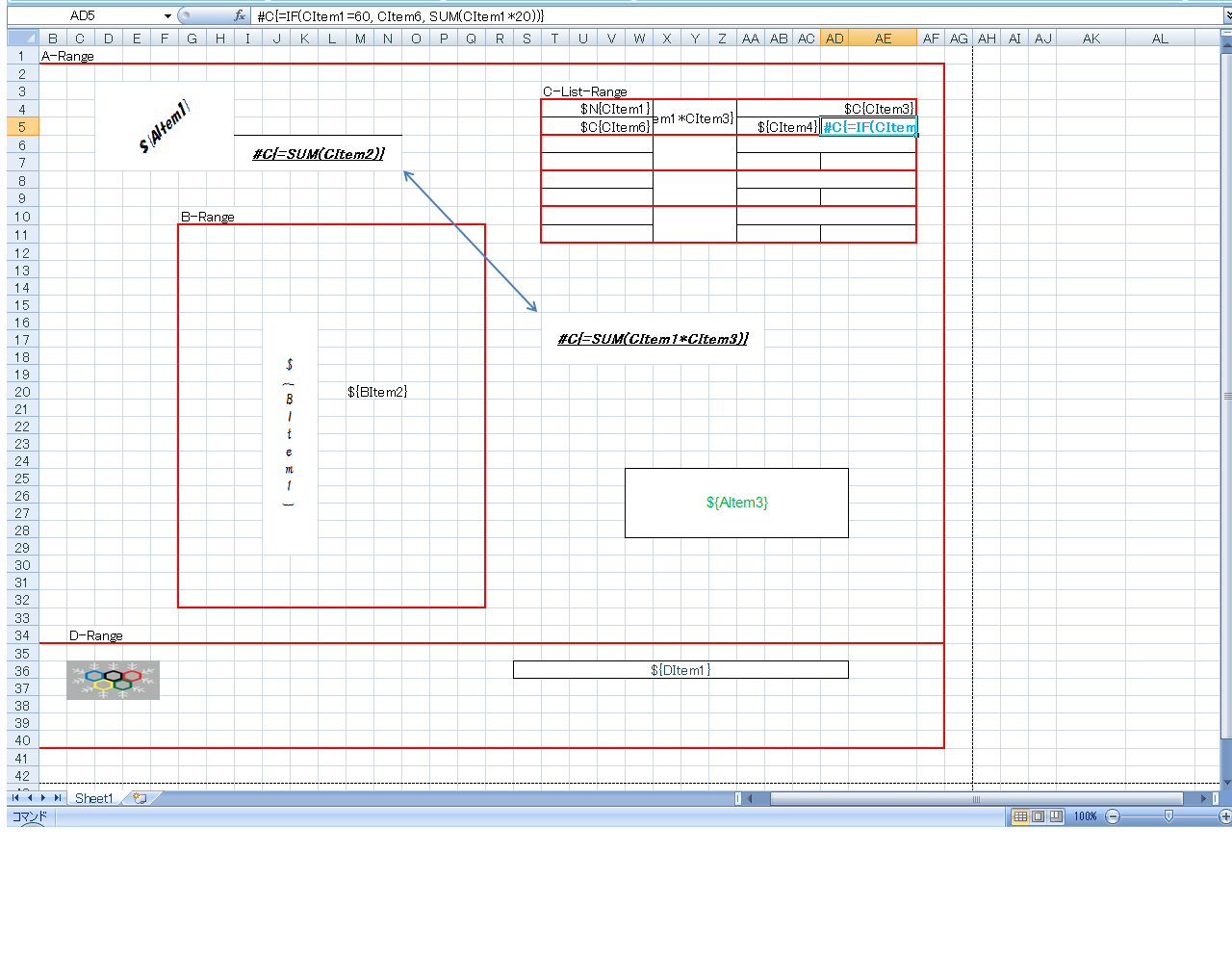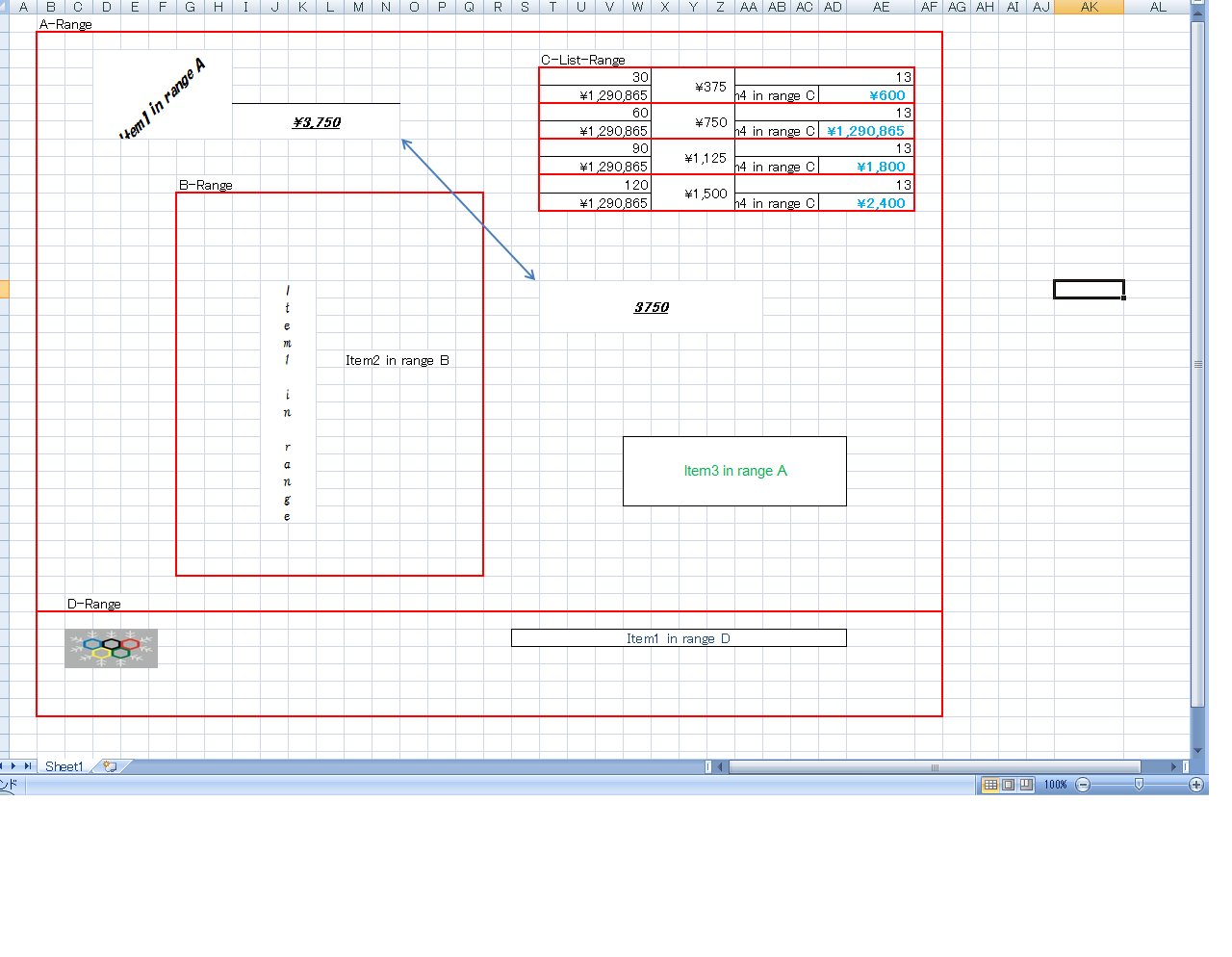# OpenXML Spreadsheet, how to copy and insert specified rows

We usually use OpenXML spreadsheet to create excel document and report. For some document and report, sometimes we hope copy and insert some rows or columns automatically. For example , we want to output a list data into excel worksheet whose element has 3 formatted rows including some merge cells etc. If list’s size is 10, we hope copy 3 formatted rows of first element 10 times and insert copied rows below the first element rows automatically.

In OpenXML spreadsheet, when you copy some rows and insert copied rows into destination, there are many works to do. First, in the copied rows, maybe there are some merge cells, data validate list ( pull down cells), formulas, drawings, charts. You must change the row’s index number to the inserted rows. Then the row’s index number for objects below in the destination must be changed. These objects include normal cells, merge cells, data validate list, formulas, drawings, charts etc.

The following is part of code to explain how to implement copy and insert. About the complete examples, please Download ExcelTDF Package

```        /// <summary>
/// Copy the part of rows from srcRowFrom to srcRowTo between srcColFrom and srcColTo.
/// The row index, whose  column is between column srcColFrom and srcColTo, and whose
/// row is below the destRowFrom, will be changed after cloned cells added into worksheet.
/// </summary>
/// <param name="workbookPart"><see cref="WorkbookPart"/></param>
/// <param name="sheetIndex">worksheet index</param>
/// <param name="srcRowFrom">source from row index</param>
/// <param name="srcRowTo">source to row index</param>
/// <param name="destRowFrom">destination from row index</param>
/// <param name="copyTimes">the times to be copied</param>
/// <param name="srcColFrom">limitation of column left</param>
/// <param name="srcColTo">limitation of column right</param>
public static void CopyRowRange(WorkbookPart workbookPart, int sheetIndex,
int srcRowFrom, int srcRowTo, int destRowFrom, int copyTimes, int srcColFrom, int srcColTo)
{
//only support copy down
if (copyTimes <= 0 || srcRowTo < srcRowFrom || destRowFrom < srcRowFrom) return;
int destRowFromBase = destRowFrom;

//Get the source sheet to be copied
WorksheetPart worksheetPart = GetWorksheetPart(workbookPart, sheetIndex);
SheetData sheetData = worksheetPart.Worksheet.GetFirstChild<SheetData>();

//get cells to be cloned according to the specified rows and columns
IList<Cell> cells = sheetData.Descendants<Cell>().Where(c =>
GetRowIndex(c.CellReference) >= srcRowFrom &&
GetRowIndex(c.CellReference) <= srcRowTo &&
GetCellColIndex(c.CellReference) >= srcColFrom &&
GetCellColIndex(c.CellReference) <= srcColTo).ToList<Cell>();

//no cells to be cloned
if (cells.Count() == 0) return;

//after cloned, the index of rows from destRowFrom should be changed
//diff rows between srcColFrom and srcColTo
int copiedRowCount = srcRowTo - srcRowFrom + 1;

//move row index between srcColFrom and srcColTo that the row index greater or equal to
//destRowFrom
MoveRowIndex(workbookPart, sheetIndex, destRowFrom - 1, copiedRowCount * copyTimes, srcColFrom, srcColTo);

//temporary array of changed row index
IList<int> changeRowIndexs = new List<int>();

//cloned cells, row index/cells
IDictionary<int, IList<Cell>> clonedCells = null;

//formula cells in cloned cells
IList<Cell> formulaCells = new List<Cell>();

//cloned related rows for speed
IList<Row> cloneRelatedRows = new List<Row>();

for (int i = 0; i < copyTimes; i++)
{
//next copy col index
destRowFrom = destRowFromBase + copiedRowCount * i;
// changed column index
int changedRows = destRowFrom - srcRowFrom;

//add change column index to list
//clear formula cells
formulaCells.Clear();

//1: cloned cells, row index/cell list
clonedCells = new Dictionary<int, IList<Cell>>();

foreach (Cell cell in cells)
{
Cell newCell = (Cell)cell.CloneNode(true);
int[] indexs = GetCellIndex(cell.CellReference);
//change row index of cloned cell
int rowIndex = indexs + changedRows;
newCell.CellReference = GetColumnName(cell.CellReference) + rowIndex.ToString();

IList<Cell> rowCells = null;
if (clonedCells.ContainsKey(rowIndex))
rowCells = clonedCells[rowIndex];
else
{
rowCells = new List<Cell>();
}

//if is formula cell
if (newCell.CellFormula != null && newCell.CellFormula.Text.Length > 0)
{
}
}

//add cloned cell into row
foreach (int rowIndex in clonedCells.Keys)
{
Row row = sheetData.Elements<Row>().Where(r => r.RowIndex == rowIndex).FirstOrDefault();
//if no this row
if (row == null)
{
row = new Row() { RowIndex = (uint)rowIndex };
//find insert position
Row refRow = sheetData.Elements<Row>().Where(r => r.RowIndex > rowIndex).OrderBy(r => r.RowIndex).FirstOrDefault();
if (refRow == null)
sheetData.AppendChild<Row>(row);
else
sheetData.InsertBefore<Row>(row, refRow);
}
row.Append(clonedCells[rowIndex].ToArray());

//add into clone related rows
}

//2: processing copied range's formula
ChangeFormulaRowNumber(formulaCells, changedRows);

//3: copy drawings in range
CopyDrawingsInRange(worksheetPart, srcRowFrom, srcRowTo, srcColFrom, srcColTo, destRowFrom, -1);
}

foreach (Row row in cloneRelatedRows)
{
// sort by column name
IList<Cell> cs = row.Elements<Cell>().OrderBy(c => GetCellColIndex(c.CellReference.Value)).ToList<Cell>();
row.RemoveAllChildren();
row.Append(cs.ToArray());
}

//2: process merge cell in cloned rows
MergeCells mcells = worksheetPart.Worksheet.GetFirstChild<MergeCells>();
if (mcells != null)
{
IList<MergeCell> newMergeCells = new List<MergeCell>();
IEnumerable<MergeCell> clonedMergeCells = mcells.Elements<MergeCell>().
Where(m => MergeCellInRange(m, srcRowFrom, srcRowTo, srcColFrom, srcColTo)).ToList<MergeCell>();
foreach (MergeCell cmCell in clonedMergeCells)
{
foreach (int changedRows in changeRowIndexs)
{
MergeCell newMergeCell = CreateChangedRowMergeCell(cmCell, changedRows);
}
}
uint count = mcells.Count.Value;
mcells.Count = new UInt32Value(count + (uint)newMergeCells.Count);
mcells.Append(newMergeCells.ToArray());
}

//3: process datavalidate list
IDictionary<string, DataValidation> validates = GetDataValidatesInRange(worksheetPart, srcRowFrom, srcRowTo, srcColFrom, srcColTo);
foreach (string cellname in validates.Keys)
{
foreach (int changedRows in changeRowIndexs)
{
}
}
}
```

# ExcelTDF, create excel report by metadata quickly

ExcelTDF provides very simple way to create complicated excel report. ExcelTDF is able to process row range, column range, block range and complicated range which including some child ranges such as list, list clone range, list-in-list range etc. You can define these ranges by Metadata. Let us show some examples.

1. Create a console application project: assume it is S:\project\FastExcelReport.

2. Set ExcelTDF configuration as following.

2.1. Add ExcelTDF repository configuration file Repository.config into your project. The content of Repository.config is shown as following

```<?xml version="1.0" encoding="utf-8" ?>
<objects xmlns="http://www.springframework.net">

<object id="mydemo" type="JanaBiz.OpenXml.Repository.FileSystemRepositoryManager, JanaBiz.OpenXml">
<property name="RepositoryName" value="mydemo"/>
<property name="RepositoryUri" value="../Templates"/>
</object>
</objects>
```

By the above configuration, your template and template range configuration file are placed in the directory of ../Templates/mydemo (RepositoryName) which is related the executable directory bin. The template file name *.xlsx and template range configuration file *.xml must have the same name, and this name is also their Template ID. ExcelTDF will search all templates and template range configuration files in root repository and subdirectories. When you use metadata, no template range configuration file is needed

3. Design your template, here named MetadataTest.xslx, and save it into the specified path of repository configured above S:\project\FastExcelReport\Templates\mydemo\MetadataTest.xlsx4. Create your data model according to the template ranges.

4.1. Add reference assemblies
. JanaBiz.OpenXml.dll
. DocumentFormat.OpenXml.dll
. log4net.dll
. Spring.Core.dll

4.2. Create data model class as following

```using System;
using System.Collections.Generic;
using System.Linq;
using System.Text;
using JanaBiz.OpenXml;

//define class for each range
namespace FastExcelReport
{
/// <summary>
/// Assume A-Range is root range
/// </summary>
[ExcelRange("A-Range", "A1", "AF40", "BlockRangeService")]
{
{
for (int i = 0; i < 4; i++)
{
{
Item1 = (i + 1) * 30
});
}
}

[Excel("AItem1")]
public string Item1 = "Item1 in range A";

[Excel("AItem3")]
public string Item3 = "Item3 in range A";

[ExcelRange("B-Range", "G11", "Q32", "BlockRangeService")]
public BRange bRange = new BRange();

/// <summary>
/// The range is defined by first element area
/// </summary>
[ExcelRange("C-Range", "T4", "AE5", "RowListRangeService", PageSize = 4)]
public IList<CRange> cRangeList = new List<CRange>();

[ExcelRange("D-Range", "B35", "AF40", "BlockRangeService")]
public DRange dRange = new DRange();
}

/// <summary>
/// The data model of B-Range
/// </summary>
public class BRange
{
[Excel("BItem1")]
public string Item1 = "Item1 in range B";

[Excel("BItem2")]
public string Item2 = "Item2 in range B";

}

/// <summary>
/// The data model of C-Range
/// </summary>
public class CRange
{
[Excel("CItem1")]
public int Item1 = 300;

[Excel("CItem2")]
public double Item2;  //save the result of sum

[Excel("CItem3")]
public double Item3 = 12.5;

[Excel("CItem4")]
public string Item4 = "Item4 in range C";

[Excel("CItem6")]
public long Item6 = 1290865;
}

/// <summary>
/// The data model of D-Range
/// </summary>
public class DRange
{
private string item1 = "Item1 in range D";

[Excel("DItem1")]
public string Item1
{
get { return item1; }
set { item1 = value; }
}
}
}
```

5. Add following template processing code into your program. That is over

```using System;
using System.Collections.Generic;
using System.Linq;
using System.Text;
using System.IO;
using System.Reflection;

using JanaBiz.OpenXml.Template;
using JanaBiz.OpenXml.Handler;
using JanaBiz.OpenXml.Data;
using JanaBiz.OpenXml.Context;
using JanaBiz.OpenXml.Repository;

//load configuration of repository
[assembly: RegisterRepository("FastExcelReport.Repository.config")]

namespace FastExcelReport
{
class Program
{
static void Main(string[] args)
{

//            OpenXmlContext.RegisterRepository("FastExcelReport.Repository.config");

IOpenXmlHandler handler = new OpenXmlHandler();

//tell ExcelTDF which ITemplateService is used to
//get template file and template range configuration
handler.GetTemplateService<LocalTemplateService>();

//set template id to identify which template is used

//tell ExcelTDF which IDataProviderManager and
//what data provider is used
handler.DataProviderManager = new DataModelProviderManager(

handler.ParseTemplate(false);

handler.ParseWorkbook(null);

//PLEASE REPLACE with your file stream to get result document
FileStream fs = new FileStream(@"c:\temp\metadatatest-result.xlsx", FileMode.Create);

//write parsed document into response stream
handler.WriteParsedDocument(fs);
fs.Close();

//release some resource
handler.Close();
}
}
}
```

The line of

```[assembly: RegisterRepository("FastExcelReport.Repository.config")]
```

is used to load Repository.config file.

The result of pecessed template is show as following.